Next: Electric Dipoles Up: Electrostatic Fields Previous: Electric Scalar Potential

# Electrostatic Energy

Consider a collection of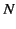static point charges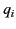, located at position vectors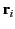, respectively (where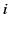runs from 1 to). Let us determine the electrostatic energy stored in such a collection. In other words, let us calculate the amount of work required to assemble the charges, starting from an initial state in which they are all at rest and very widely separated.

The work we would have to do against electrical forces in order to slowly move a charge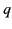from point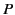to point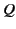is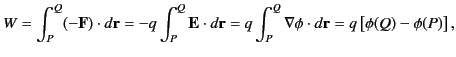(164)

where use has been made of Equations (149) and (154). Note that to move the charge we have to exert on it a force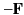, where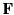is specified in Equation (149), in order to counteract the force exerted by the electric field. Recall that the scalar potential field generated by a point charge, located at position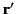, is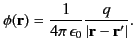(165)

Let us build up our collection of charges one by one. It takes no work to bring the first charge from infinity, because there is no electric field to fight against. Let us clamp this charge in position at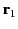. In order to bring the second charge into position at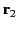, we have to do work against the electric field generated by the first charge. According to Equations (164) and Equations (165), this work is given by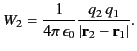(166)

Let us now bring the third charge into position. Because electric fields and scalar potentials are superposable, the work done while moving the third charge from infinity to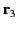is simply the sum of the works done against the electric fields generated by charges 1 and 2 taken in isolation: that is,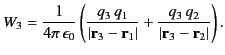(167)

Thus, the total work done in assembling the three charges is given by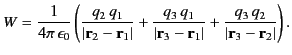(168)

This result can easily be generalized tocharges: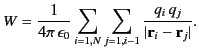(169)

The restriction that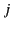must be less thanmakes the above summation rather cumbersome. If we were to sum without restriction (other than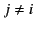) then each pair of charges would be counted twice. It is convenient to do just this, and then to divide the result by two. Thus, we obtain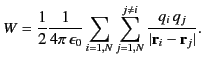(170)

This expression specifies the electrostatic potential energy of a collection of point charges. We can think of this energy as the work required to bring stationary charges from infinity and assemble them in the required formation. Alternatively, it is the kinetic energy that would be released if the collection were dissolved, and the charges returned to infinity. Let us investigate how the potential energy of a collection of electric charges is stored.

Equation (170) can be written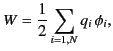(171)

where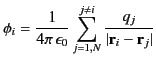(172)

is the scalar potential experienced by theth charge due to the other charges in the distribution.

Let us now consider the potential energy of a continuous charge distribution. It is tempting to write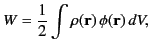(173)

by analogy with Equations (171) and (172), where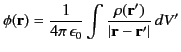(174)

is the familiar scalar potential generated by a continuous charge distribution of charge density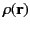. Let us try this ansatz out. We know from Equation (159) that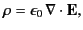(175)

so Equation (173) can be written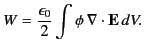(176)

Making use of the vector identity,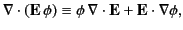(177)

as well as the fact that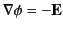, we obtain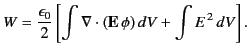(178)

Application of the divergence theorem gives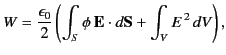(179)

where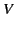is some volume that contains all of the charges, and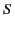is its bounding surface. Let us assume thatis a sphere, centered on the origin, and let us take the limit in which the radius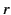of this sphere goes to infinity. We know that, in general, the electric field a large distancefrom a bounded charge distribution looks like the field of a point charge, and, therefore, falls off like. Likewise, the potential falls off like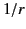. However, the surface area of a sphere of radiusincreases like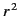. Hence, it is clear that, in the limit as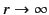, the surface integral in Equation (179) falls off like, and is consequently zero. Thus, Equation (179) reduces to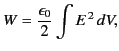(180)

where the integral is over all space. This expression implies that the potential energy of a continuous charge distribution is stored in the electric field generated by the distribution, assuming that this field possesses the energy density (see Section 1.9)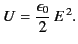(181)

We can easily check that Equation (180) is correct. Suppose that we have an amount of chargethat is uniformly distributed within a sphere of radius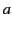, centered on the origin. Let us imagine building up this charge distribution from a succession of thin spherical layers of infinitesimal thickness. At each stage, we gather a small amount of charge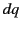from infinity, and spread it over the surface of the sphere in a thin layer extending fromto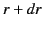. We continue this process until the final radius of the sphere is. Ifis the sphere's charge when it has attained radiusthen the work done in bringing a chargeto its surface is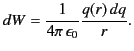(182)

This follows from Equation (166), because the electric field generated outside a spherical charge distribution is the same as that of a point chargelocated at its geometric center (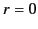). If the uniform charge density of the sphere is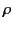then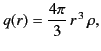(183)

and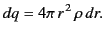(184)

Thus, Equation (182) becomes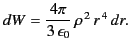(185)

The total work needed to build up the sphere from nothing to radiusis plainly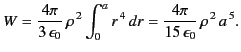(186)

This can also be written in terms of the total charge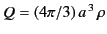as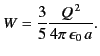(187)

Now that we have evaluated the potential energy of a spherical charge distribution by the direct method, let us work it out using Equation (180). We shall assume that the electric field is both radial and spherically symmetric, so that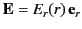. Application of Gauss' law,(188)

whereis a sphere of radius, centered on the origin, gives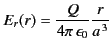(189)

for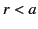, and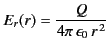(190)

for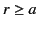. Equations (180), (189), and (190) yield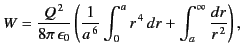(191)

which reduces to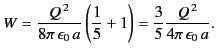(192)

Thus, Equation (180) gives the correct answer.

The reason that we have checked Equation (180) so carefully is that, on close inspection, it is found to be inconsistent with Equation (171), from which it was supposedly derived. For instance, the energy given by Equation (180) is manifestly positive definite, whereas the energy given by Equation (171) can be negative (it is certainly negative for a collection of two point charges of opposite sign). The inconsistency was introduced into our analysis when we replaced Equation (172) by Equation (174). In Equation (172), the self-interaction of theth charge with its own electric field is specifically excluded, whereas it is included in Equation (174). Thus, the potential energies (171) and (180) are different because in the former we start from ready-made point charges, whereas in the latter we build up the whole charge distribution from scratch. Consequently, if we were to calculate the potential energy of a point charge distribution using Equation (180) then we would obtain the energy (171) plus the energy required to assemble the point charges. However, the latter energy is infinite. To see this, let us suppose, for the sake of argument, that our point charges actually consist of charge uniformly distributed in small spheres of radius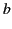. According to Equation (187), the energy required to assemble theth point charge is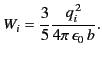(193)

We can think of this as the self-energy of theth charge. Thus, we can write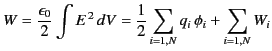(194)

which enables us to reconcile Equations (171) and (180). Unfortunately, if our point charges really are point charges then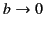, and the self-energy of each charge becomes infinite. Thus, the potential energies specified by Equations (171) and (180) differ by an infinite amount. We are forced to the conclusion that the idea of locating electrostatic potential energy in the electric field is inconsistent with the existence of point charges. One way out of this difficulty would be to say that elementary charges, such as electrons, are not points objects, but instead have finite spatial extents. Regrettably, there is no experimental evidence to back up this assertion. Alternatively, we could say that our classical theory of electromagnetism breaks down on very small length-scales due to quantum effects. Unfortunately, the quantum mechanical version of electromagnetism (which is called quantum electrodynamics) suffers from the same infinities in the self-energies of charged particles as the classical version. There is a prescription, called renormalization, for steering round these infinities, and getting finite answers that agree with experimental data to extraordinary accuracy. However, nobody really understands why this prescription works.Next: Electric Dipoles Up: Electrostatic Fields Previous: Electric Scalar Potential
Richard Fitzpatrick 2014-06-27